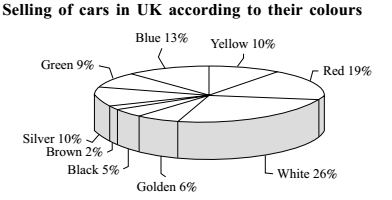## Mixed Graph

#### Data Interpretation

Direction: Study the chart and give the answers of following questions.1. Cars of which color when increased by 2 % and then combined with that of red cars will make 30 % of the total?

1. From above given chart ,
selling of red colours' cars = 19%
Then , 30 - 19 = 11%
Required answer = 11 - 2 = 9% ( green )
The correct option is E .

##### Correct Option: E

Given :- total percent of the make cars = 30%
selling of red colours' cars = 19%
Then , 30 - 19 = 11%
when increased by two percent ,
Required answer = 11 - 2 = 9% ( green )

1. Cars of which color are 13% less popular than white colored cars?

1. As per above given chart ,we have
selling of white colours' cars = 26%
Since Cars of golden colour are 20% less popular than white coloured cars.

##### Correct Option: A

As per above given chart ,we have
selling of white colours' cars = 26%
Since Cars of blue colour are 13% less popular than white coloured cars.
Required answer = 26 - 13 = 13% ( Blue )

1. 50% of all the cars consisted of which colors of car?

1. From above given chart ,
selling of white colours' cars = 26%
selling of Golden colours' cars = 6%
selling of Blue colours' cars = 13%
selling of Black colours' cars = 5%
Since ,50% of all the cars consist of White, Golden, Blue and Black colours of cars .

##### Correct Option: C

From above given chart ,
selling of white colours' cars = 26%
selling of Golden colours' cars = 6%
selling of Blue colours' cars = 13%
selling of Black colours' cars = 5%
Since , 50% of all the cars consist of White, Golden, Blue and Black colours of cars .
Required answer = 26 + 6 + 13 + 5 = 50%

1. If in a certain period the total production of all cars was 95400 then how many more blue cars were sold than green?

1. Required answer = No. of blue cars sold - No. of Green cars sold

##### Correct Option: D

From above given chart ,we have
total production of all cars = 95400

 No. of blue cars sold = 13 x 95400 = 12402 100

 No. of Green cars sold = 9 x 95400 = 8586 100

Required answer = No. of blue cars sold - No. of Green cars sold
= 12402 - 8586 = 3816

1. Cars of which color are 20% less popular than white colored cars?

1. From above given chart ,
selling of white colours' cars = 26%
Since Cars of golden colour are 20% less popular than white coloured cars.

##### Correct Option: B

From above given chart ,
selling of white colours' cars = 26%
Since Cars of golden colour are 20% less popular than white coloured cars.
Required answer = 26 - 20 = 6%